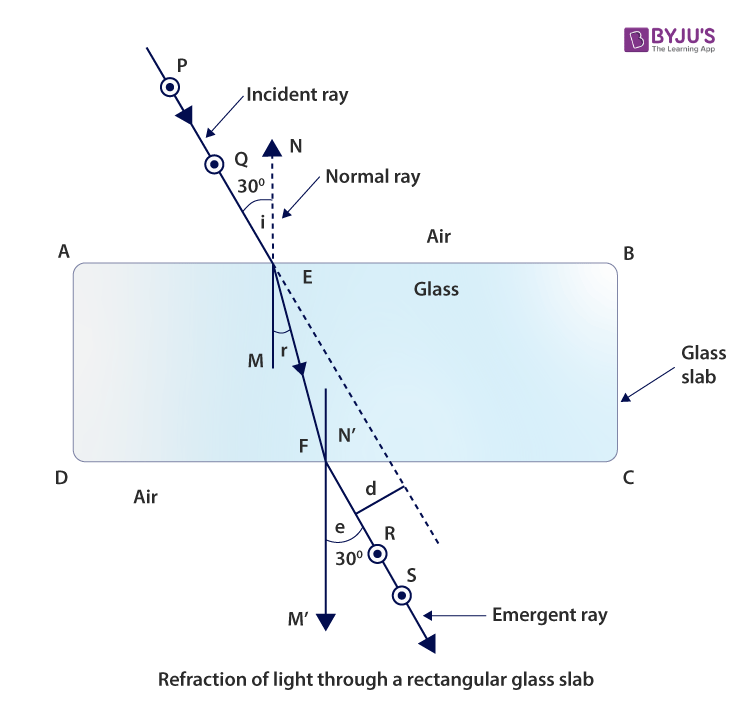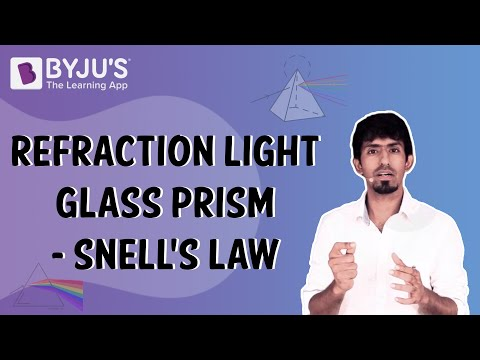Jet Set Go! All about Aeroplanes Jet Set Go! All about Aeroplanes

# Tracing Path of a Ray of Light Passing Through a Glass Slab

Refraction is the property of light due to which it bends its path while travelling from one medium to another. The law of refraction is also known as Snell’s law which states that the ratio of the sine of the angle of incidence to the sine of the angle of refraction is equal to constant. Below is an experiment to trace the path of a light ray passing through the rectangular glass slab.

## Aim

To trace the path of a ray of light passing through a rectangular glass slab for different angles of incidence. Measure the angle of incidence, angle of refraction, and angle of emergence, and interpret the result.

## Theory

#### What are the laws of refraction?

Following are the laws of refraction:

• The incident ray, the normal at the point of incidence, and the refracted ray lie in the same plane.
• Snell’s law states that the ratio of the sine of the angle of incidence to the sine of the angle of refraction is constant.
$$\begin{array}{l}\frac{sin\;i}{sin\;r}=constant\end{array}$$

#### What is refraction of light?

The refraction of light is a property of light due to which it changes its path when it passes from one medium to the other.

#### What is lateral displacement?

Lateral displacement is defined as the perpendicular shift in the path of light when it emerges out from the refracting medium.

## Materials Required

1. A drawing board
2. 4-6 all pins
3. White sheet of paper
4. Rectangular glass slab
5. A protractor
6. A scale
7. A pencil
8. Thumb pins

## Procedure

1. Fix a white sheet on the soft drawing board using thumb pins.
2. Place the glass slab at the centre of the white paper and draw its outline boundary using a sharp pencil.
3. Let ABCD be the rectangular figure obtained by drawing.
4. Mark a point E on AB and draw a perpendicular EN and label it as a normal ray.
5. Draw one angle of 30° with the help of protractor with EN. Fix pins at P and Q at 4-5 cm on the ray that is obtained by the angle.
6. Place the glass slab on the rectangular figure ABCD.
7. To fix R and S, see through the glass slab from side CD, such that when seen through the glass slab, all the pins P, Q, R, and S should lie in a straight line.
8. Draw small circles around the pins P, Q, R and S and remove the pins.
9. Remove the glass slab.
10. Join points R and S such that it meets CD at point F. Draw a perpendicular N’M’ to CD at point F.
11. Using a pencil, join the points E and F.
12. Measure the angles formed at AB and CD, i.e, the incident angle, refracted angle, and emergent angle.
13. The lateral displacement is obtained by extending the ray PQ in a dotted line which is parallel to ray FRS.
14. Measure the lateral displacement.
15. Repeat the same procedure for angles 45° and 60°.

## Ray Diagram## Observation Table

 Sl.no Angle of incidence ∠i = ∠PEN Angle of refraction ∠r = ∠MEF Angle of emergence ∠e = ∠SFM’ ∠i – ∠e ∠PEN – ∠SFM’ 1 30° 28° 30° 0° 2 45° 43° 44.8° 0.2° 3 60° 56° 59.8° 0.2°

Due to human error the value of i – ∠e may not be equal to zero.

## Conclusion

1. The angle of incidence and the angle of emergence are almost equal.
2. As the light is traveling from rarer to denser optical medium, the angle of refraction will be lesser than the angle of incidence.
3. For different angles of incidence, the lateral displacement will remain the same.
4. The light will bend towards the normal when it travels from an optically rarer medium to an optically denser medium.

## Precautions

1. The rectangular glass slab used should have perfectly smooth faces.
2. The drawing board should be soft so that pins can be easily fixed on it.
3. The angle of incidence should lie between 30° and 60°.
4. All pins base should be in a straight line.
5. The distance between the pins P and Q or the pins R and S, about 5 cm gap should be maintained.
6. Using a sharp pencil, draw thin lines.
7. The quality of the protractor should be good.
8. The placement of the protractor should be correct to get correct measurements.
9. The perpendiculars should be drawn with care.

## Sources of Error

1. There shouldn’t be any air bubbles in the glass slab.
2. The measurements done by the protractor should be accurate.

## Viva Questions

Q1. How is the angle of incidence related to the angle of emergence?
Ans: The angle of incidence is equal to the angle of emergence or may differ by very small amount due to human error.

Q2. What should be the angle of incidence for the above experiment?
Ans: The angle of incidence required for the above experiment is between 30° to 60°.

Q3. On which condition does the light not deviate during the refraction of light?
Ans: When the light ray is perpendicular to the refracting surface, there is no deviation in the light ray.

Q4. Which property of light causes the rainbow formation?
Ans: The refraction of light causes the rainbow formation due to tiny water droplets in the atmosphere.

Q5. On which condition will the emergent and incident rays be parallel?
Ans: When the angle of incidence is equal to the angle of the emergent ray, the emergence and incident ray will be parallel.